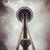# Exp function to get antilog

6890
3
10-09-2014 10:50 AMOccasional Contributor III

I am trying to take some result values and log transform them with the field calculator, then krig them to a surface - I found this in the help regarding the log function:

"field calculator uses the natural Log not Log10(x)

try;

Log ( [Area] ) / Log ( 10 )"

I did that and it seemed to work, but how do I calculate the antilog or exp back correctly of the surface, I can't seem to get back to values that correlate to my original data (which ranges from 0.00001 to 0.003047) - I run:

Exp("log_krig")

in the raster calculator and end up with values of 0.00252001 to 0.0755711...I'm wondering if there's a reverse of the above trick to get the antilog of my surface somehow (i.e. get back from Log transformed data to original)? My mathematics are not strong...thanks for any help!

1 Solution

Accepted SolutionsbyMVP Esteemed Contributor

Natural log   Ln    This example calculates the natural logarithm (base e) of the input raster values

Exp                      This example calculates the base e exponential of the input raster values,

Is this the pairing you are looking for?  there is Log10, Ln, Log2  and Exp10, Exp, Exp2

3 RepliesbyMVP Esteemed Contributor

Natural log   Ln    This example calculates the natural logarithm (base e) of the input raster values

Exp                      This example calculates the base e exponential of the input raster values,

Is this the pairing you are looking for?  there is Log10, Ln, Log2  and Exp10, Exp, Exp2Occasional Contributor III

Wow, somehow I just did not see the exp10 in the raster calculator list the first time - thanks Dan!byMVP Esteemed Contributor

No problem...the terms differ depending upon which software you are using...wait until you get into python...